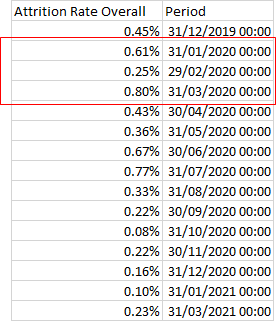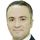cancel
Showing results for
Did you mean:Helper II

## Excel Formula into Dax

Hi all, I am trying to calculate Projected Attrtion Rate using the formula

Projected Attrition Rate = Cumulative Attrition Rate/# Cumulative Months in the Period * 12 months

i.e. : [(Jan+Feb+Mar)/3]*12 = [(0.61%+0.25%+0.8%)/3]*12

The Attrition Rate Overall  use in the table is:

Need some help to apply the formula into powerbi. Any help or reference? Thanks!1 ACCEPTED SOLUTIONSolution Specialist

Then you can iterate over a summarized calendar with your measure as follows:

``````Attrition Rate =
CALCULATE (
AVERAGEX (
SUMMARIZE ( 'Calendar', 'Calendar'[Date] ),
"AttritionRate", [Attrition Rate]
),
[AttritionRate]
),
DATESYTD ( 'Calendar'[Date] )
) * 12``````
3 REPLIES 3Solution Specialist

The way we can write the synatx could vary depending on your data structure. I assume that you have a calendar table.

``````Attrition Rate =
CALCULATE (
DATESYTD ( 'Calendar'[Date] )
) * 12``````Helper II

However, my Attrition Rate is actually a measure. Which I cannot apply averagex on it. Any advice on this?Solution Specialist

Then you can iterate over a summarized calendar with your measure as follows:

``````Attrition Rate =
CALCULATE (
AVERAGEX (
SUMMARIZE ( 'Calendar', 'Calendar'[Date] ),
"AttritionRate", [Attrition Rate]
),
[AttritionRate]
),
DATESYTD ( 'Calendar'[Date] )
) * 12``````